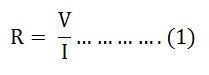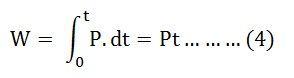# Resistance

Resistance is the property of a material by virtue of which it opposes the flow of electrons through the material. It restricts the flow of the electron through the material. It is denoted by (R) and is measured in ohms (Ω).

When the voltage is applied across the resistor the free electrons start accelerating. These moving electrons collide with each other and hence opposes the flow of electrons. The opposition of electrons is known as the resistance. The heat is generated when the atom or molecules are colliding with each other.

Contents:

Explanation:

The resistance of any material depends on two factors

• The shape of the material
• Material type (what material it is made up of)

Quantitatively it is obtained by Ohm’s Law, as the resistance offered by the material when a current of I ampere flows through it with a potential difference of (V) volts across the material. It is given by the equation shown belowWhere R is the resistance, V is the voltage, and I is the current in the circuit.

It is clear from the above equation (1) that resistance is directly proportional to the voltage and inversely proportional to the current of the circuit. It is also given asWhere,

• R is the resistance of any conductor or material measured in ohms
• ρ is the resistivity of the material and is measured in ohms meter
• l is the length of the material or conductor in meters
• A is the conductor’s cross-sectional area in meter square

The resistance of any conducting material is directly proportional to the length of the conductor and inversely proportional to the conductor’s cross-sectional area.

Resistivity (ρ) is defined as the ability of the conductor or the material to oppose the electric current. The resistance of any conductor is measured by the instrument known as Ohmmeter.

The collision of atoms with the free electrons causes the heat to develop when an electric current flows through any conductor or material. If a current of I ampere is passing through the conductor and the potential difference is V volts across the conductor, then the power absorbed by the resistor is given by the equation (3) shown belowAs we know V = IRThe energy lost in the resistance in the form of heat and is derived asPutting the value of P from the equation (3) in equation (4) we will getAs we know I = V/R, hence putting the value of I in equation (5) we will getThe above equation (6) shows the equation for energy loss in the form of heat.

### Types of Resistance

There are mainly two types of resistance

• Static Resistance

It is similar to the normal resistance of the circuit given as R=V/I. It determines the power dissipation in an electric circuit. It is also defined as the slope of the line from the origin through various points on the curve.

• Differential Resistance

It is also known as the incremental or dynamic resistance of the circuit. It is the derivative of the ratio of voltage to the current. Differential resistance is given by the formula shown below

##### Series and Parallel Resistance in the circuit

Series Resistance Circuit

If the various resistances suppose R1, R2, R3 connected together in series as shown in the figure below is termed as a series resistance circuitThe equivalent or total resistance is given by the equationParallel Resistance Circuit

The various resistances suppose R1, R2, R3 are connected in parallel with each other as shown in the circuit below is known as Parallel Resistance Circuit.The equivalent or total resistance is given by the equation